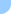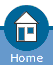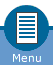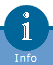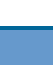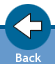Glossary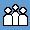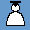Amplitude: The maximum displacement from the state of equilibrium during vibration. In the case of waves within wave-propagation, it is the maximum displacement from the state of equilibrium of the oscillating particles. Symbol: A Physical unit: 1 metre (1 m)Frequency: It shows the number of oscillations performed in one second. Symbol: f Physical unit: 1 Hertz (1 Hz = 1/second)   Phase: Such a state of the body carrying out the harmonic oscillation, which is determined by the part of the periodical movement in which the body is at a given moment.   Propagation speed: The propagation speed of the oscillation phase is defined as the propagation (phase)speed of the wave. Symbol: c Physical unit: 1 metre/second (1 m/s)   Wavelength:The particles at a big distance from the wave-source start their oscillatory movement later than those closer to the source. Consequently, they are in a different phase of oscillation at a given moment. The wavelength is the distance between the two closest adjacent points in the same phase. Symbol: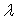Physical unit: 1 metre (1 m)